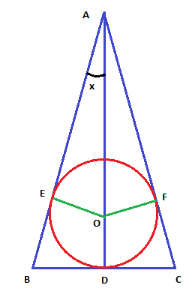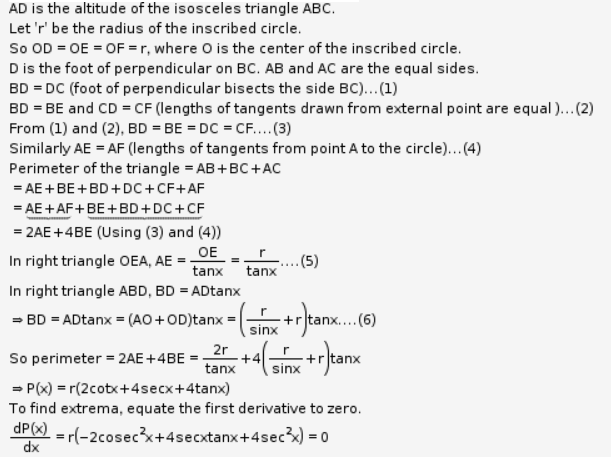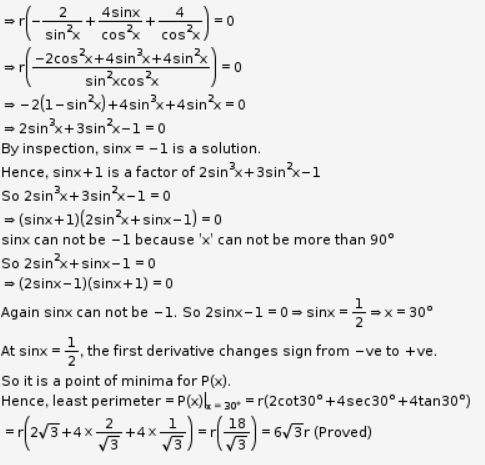# Prove that the least perimeter of an isosceles triangle in which a circle of radius r can be inscribed is 6r√3

Prove that the least perimeter of an isosceles triangle in which a circle of radius r can be inscribed is 6r√3